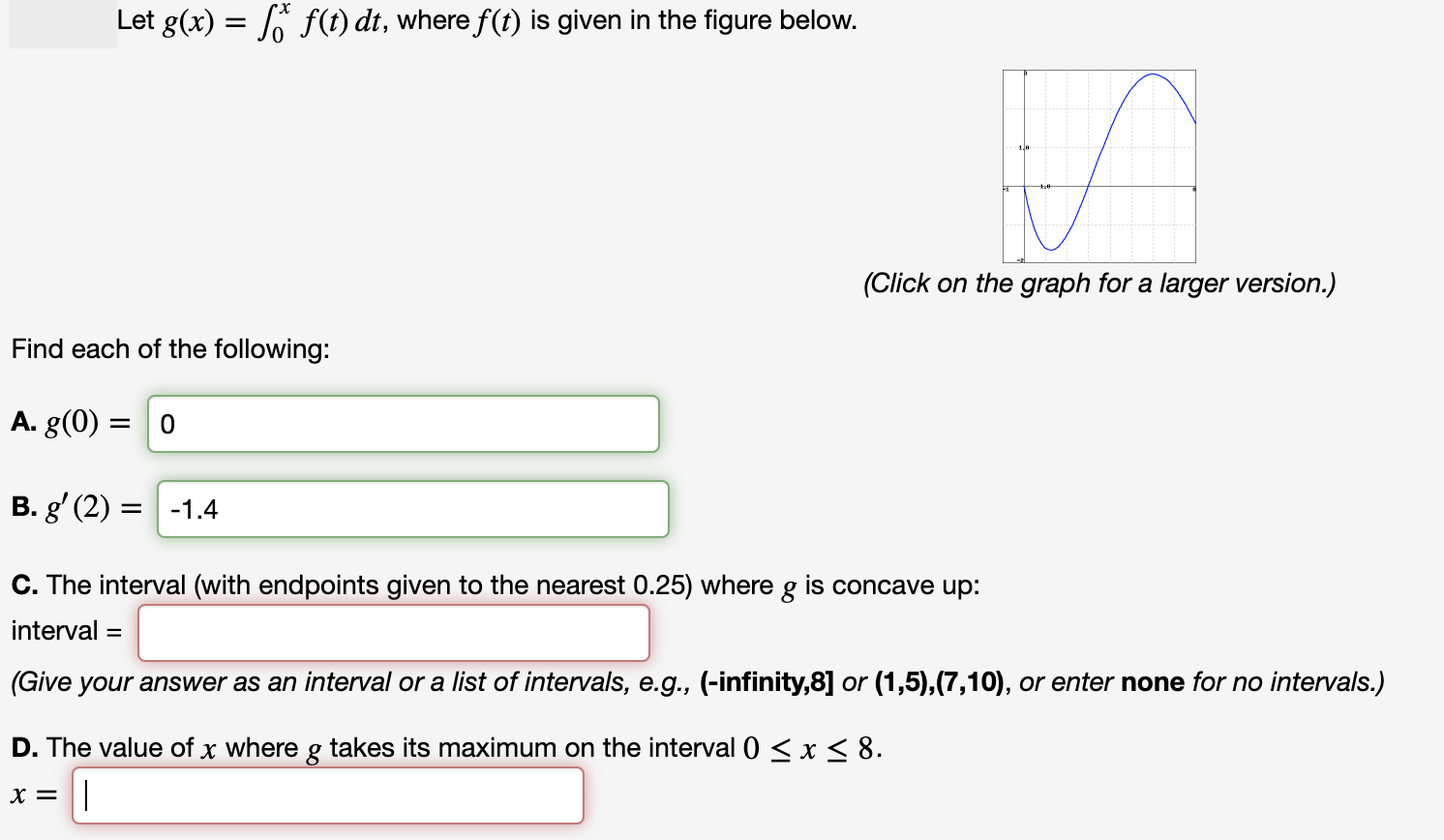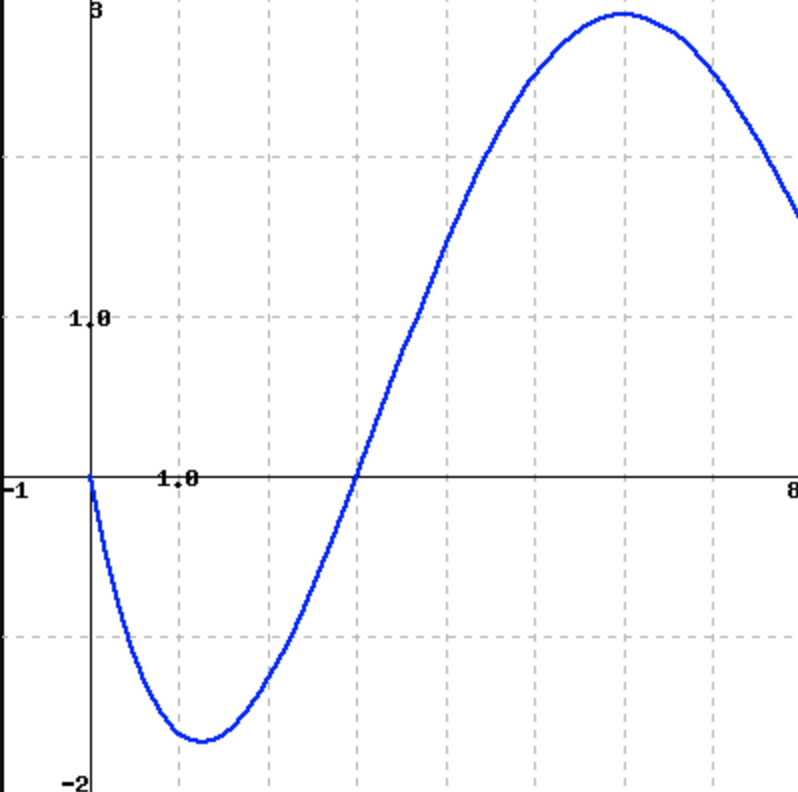# f(t) dt, where f(t) is given in the figure below.Let g(x)(Click on the graph for a larger version.)Find each of the following:A. g(0)0B. g' (2)-1.4C. The interval (with endpoints given to the nearest 0.25) where g is concave up:interval(Give your answer as an interval or a list of intervals, e.g., (-infinity,8] or (1,5),(7,10), or enter none for no intervals.)D. The value of x where g takes its maximum on the interval 0x< 8 10-1

Question
27 viewshelp_outlineImage Transcriptionclosef(t) dt, where f(t) is given in the figure below. Let g(x) (Click on the graph for a larger version.) Find each of the following: A. g(0) 0 B. g' (2) -1.4 C. The interval (with endpoints given to the nearest 0.25) where g is concave up: interval (Give your answer as an interval or a list of intervals, e.g., (-infinity,8] or (1,5),(7,10), or enter none for no intervals.) D. The value of x where g takes its maximum on the interval 0 x< 8 fullscreenhelp_outlineImage Transcriptionclose10 -1 fullscreen
check_circle

Step 1

### Want to see the full answer?

See Solution

#### Want to see this answer and more?

Solutions are written by subject experts who are available 24/7. Questions are typically answered within 1 hour.*

See Solution
*Response times may vary by subject and question.
Tagged in

### Calculus